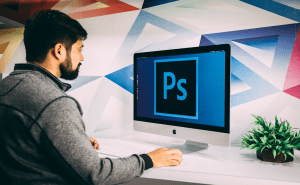# MathType screenshots

Write complex equations while using dozens of available symbols and functions.MathType 6.7 : Functions Recognized2.7.1
FREEUses OCR to convert math equations from the screen into LaTex code.2.06.10Infinite Algebra 2 app covers all typical Algebra material.3.3.0
FREEOpenOffice.org Math - Create equations and formulas for your documents.

## Popular stories

See all